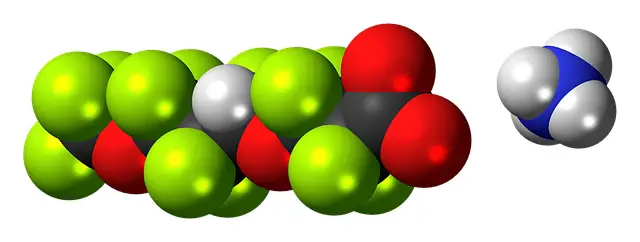PhysicsTeacher.in

High School Physics BLOG - online class notesRSS Feed

MeasurementsDifference between mole and molecule-mol or mole vs molecule

Difference between mole and molecule – mole vs molecule While studying the chapter on gas laws you must have come across the term ‘mole’ or ‘mol‘. You may have seen gram-mol as well in that chapter. I find many students confused with mole (or mol) and molecule. Here we will discuss and explain the concept ofHow does Density differ from Relative Density?

Difference between Density and Relative Density Density Density is the mass per unit volume. So if we divide the mass of a substance with its volume then we get the density of that substance. Here we get another definition of mass. Mass=volume X density.  Unit of density in SI is Kg/meter3. So if we say densityMass and Weight- are they same or different?

Mass and Weight What are Mass and Weight ? Are they same? Often we mess up with these two while discussing physics fundamentals or nymerical problems. To answer these questions we will first discuss on the concepts of mass and weight. As you get the basics of these 2 physical quantities, certainly you will be

error: Content is protected !!